# Bin Size in Matplotlib Histogram

Prerequisites: Matplotlib

A histogram is a graphical representation of the distribution of data given by the user. Its appearance is similar to Bar-Graph except it is continuous.

The towers or bars of a histogram are called bins. The height of each bin shows how many values from that data fall into that range.

Width of each bin is = (max value of data – min value of data) / total number of bins

The default value of the number of bins to be created in a histogram is 10. However, we can change the size of bins using the parameter bins in matplotlib.pyplot.hist().

### Method 1 :

We can pass an integer in bins stating how many bins/towers to be created in the histogram and the width of each bin is then changed accordingly.

Example 1 :

## Python3

 `import` `matplotlib.pyplot as plt ` ` `  `height ``=` `[``189``, ``185``, ``195``, ``149``, ``189``, ``147``, ``154``,  ` `          ``174``, ``169``, ``195``, ``159``, ``192``, ``155``, ``191``,  ` `          ``153``, ``157``, ``140``, ``144``, ``172``, ``157``, ``181``,  ` `          ``182``, ``166``, ``167``] ` ` `  `plt.hist(height, edgecolor``=``"red"``, bins``=``5``) ` `plt.show() `

Output :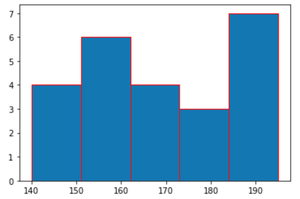Here, the bins = 5, i.e the number of bins to be created is 5. Setting bins to an integer creates bins of equal size or width. As the bin size is changed so then the bin width would be changed accordingly as :

width = (195 – 140 ) / 5 = 11

Example 2 :

## Python3

 `import` `matplotlib.pyplot as plt ` ` `  `values ``=` `[``87``, ``53``, ``66``, ``61``, ``67``, ``68``, ``62``, ` `          ``110``, ``104``, ``61``, ``111``, ``123``, ``117``, ` `          ``119``, ``116``, ``104``, ``92``, ``111``, ``90``, ` `          ``103``, ``81``, ``80``, ``101``, ``51``, ``79``, ``107``, ` `          ``110``, ``129``, ``145``, ``139``, ``110``] ` ` `  `plt.hist(values, bins``=``7``, edgecolor``=``"yellow"``, color``=``"green"``) ` `plt.show() `

Output :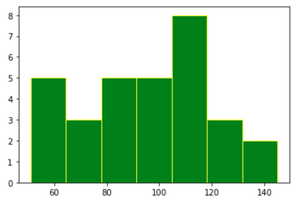In the above graph, the width of each bin is :

width = ( 145 – 51 ) / 7  = 13.4

### Method 2 :

We can also pass a sequence of int or float in the parameter bins. In which the elements of the sequence are the edges/boundaries of the bins. In this method, the bin width may vary for each bin.

Suppose a sequence [1,2,3,4,5] is assigned to bins and then the number of bins made will be 4 i.e the first bin will be [1,2) (including 1, but excluding 2) second bin will be [2,3) (including 2, but excluding 3) third bin will be [3,4) (including 3, but excluding 4). However, in the last bin [4,5] both 4 and 5 are included.

Hence, all the bins are half-open [a, b) but the last bin is closed [a, b]. For such cases, the width of each bin is equal.

If the difference between each element of the sequence assigned to bins is not equal then the width of each bin is different, hence the bin width depends on the sequence.

Example 1 : Equal bin width

## Python3

 `import` `matplotlib.pyplot as plt ` ` `  `marks ``=` `[``1``, ``2``, ``3``, ``2``, ``1``, ``2``, ``3``, ``2``,  ` `         ``1``, ``4``, ``5``, ``4``, ``3``, ``2``, ``5``, ``4``,  ` `         ``5``, ``4``, ``5``, ``3``, ``2``, ``1``, ``5``] ` ` `  `plt.hist(marks, bins``=``[``1``, ``2``, ``3``, ``4``, ``5``], edgecolor``=``"black"``) ` `plt.show() `

Output :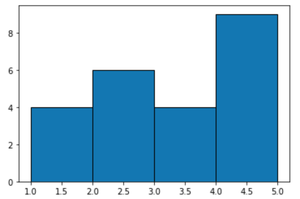Example 2 : Unequal bin width

## Python3

 `import` `matplotlib.pyplot as plt ` ` `  `data ``=` `[``189``, ``185``, ``195``, ``149``, ``189``, ``147``, ` `        ``154``, ``174``, ``169``, ``195``, ``159``, ``192``, ` `        ``155``, ``191``, ``153``, ``157``, ``140``, ``144``,  ` `        ``172``, ``157``, ``181``, ``182``, ``166``, ``167``] ` ` `  `plt.hist(data, bins``=``[``140``, ``150``, ``160``, ``175``, ``185``, ``200``], ` `         ``edgecolor``=``"yellow"``, color``=``"grey"``) ` ` `  `plt.show() `

Output: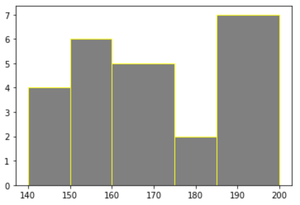### Method 3:

For passing a sequence in the bins parameter, we may also use the range function for equally distributed bins. Within range(), the starting point is the minimum of data, the endpoint is the maximum of data + the bin width mentioned, as in range(), the endpoint is not included and the step is bin width.

As the step is fixed in range(), we get equally sized bins in the histogram.

Example :

## Python3

 `import` `matplotlib.pyplot as plt ` ` `  `data ``=` `[``87``, ``53``, ``66``, ``61``, ``67``, ``68``, ``62``, ``110``, ` `        ``104``, ``61``, ``111``, ``123``, ``117``, ``119``, ``116``, ` `        ``104``, ``92``, ``111``, ``90``, ``103``, ``81``, ``80``, ``101``, ` `        ``51``, ``79``, ``107``, ``110``, ``129``, ``145``, ``128``,  ` `        ``132``, ``135``, ``131``, ``126``, ``139``, ``110``] ` ` `  `binwidth ``=` `8` `plt.hist(data, bins``=``range``(``min``(data), ``max``(data) ``+` `binwidth, binwidth), ` `         ``edgecolor``=``"yellow"``, color``=``"brown"``) ` ` `  `plt.show() `

Output :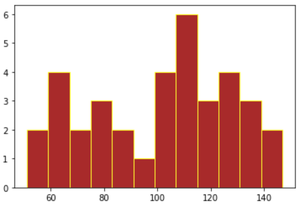Whether you're preparing for your first job interview or aiming to upskill in this ever-evolving tech landscape, GeeksforGeeks Courses are your key to success. We provide top-quality content at affordable prices, all geared towards accelerating your growth in a time-bound manner. Join the millions we've already empowered, and we're here to do the same for you. Don't miss out - check it out now!

Previous
Next